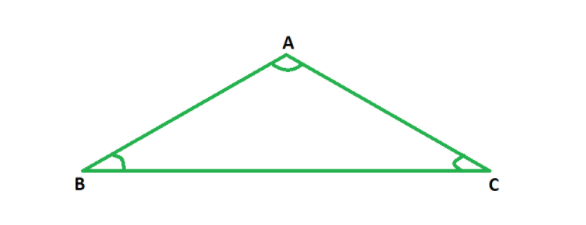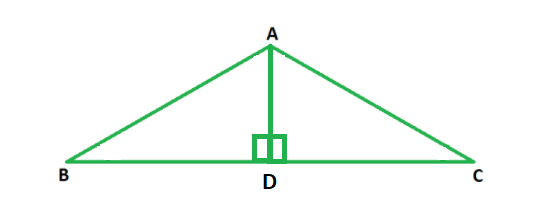Open in App
Not now

# How to find the area of an equilateral triangle when given the perimeter and height?

• Last Updated : 29 Dec, 2021

In mathematics, the proportions, measurements, dimensions, forms, shapes, and angles of different objects around us are studied under the branch called geometry. Geometry is one of the oldest branches of mathematics and has a very significant application and impact on our daily lives. We are surrounded by innumerable objects in our lives. All these objects possess a certain shape, take up significant space, can be used to store a particular amount of things in them, and can be placed as per different positions. All these factors fall under the scope of geometry. Shapes can be categorized a two- dimensional and three-dimensional.

### Equilateral Triangle

An equilateral triangle has all three sides of equal lengths and all three angles of equal measure, i.e., 60°. The figure below depicts an equilateral triangle ABC with ∠A = ∠B = ∠C = 60°.Perimeter

The perimeter of a triangle is the sum of lengths of all three sides. Since the lengths of sides of an equilateral triangle are equal,

Perimeter of an equilateral side = 3 × Side.

Area

The area of an equilateral triangle of side length a is given by the following formula:

A =### How to find the area of an equilateral triangle when given the perimeter and height?

Solution:Since perimeter of an equilateral side = 3 × Side

⇒ Side = 1/3 × Perimeter

Now, the formula A =can be applied to find the area of the given triangle.

Example: say the perimeter of an equilateral triangle is 15 cm; then

Side = 15/3 = 5 cm

Thus, area =sq. cm.

### Similar Problems

Question 1. Find the area of an equilateral triangle whose perimeter is 69 cm.

Solution:

Side = Perimeter/ 3

= 69/3

= 23 cm

Thus, area =⇒ A = 230 sq. cm.

Question 2. Find the area of an equilateral triangle whose perimeter is 180 cm.

Solution:

Side = Perimeter/ 3

= 180/3

= 60 cm

Thus, area =⇒ A = 1558.84 sq. cm.

Question 3. Find the area of an equilateral triangle whose perimeter is 60 cm.

Solution:

Side = Perimeter/ 3

= 60/3

= 20 cm

Thus, area =⇒ A = 1738.20 sq. cm.

Question 4. Find the area of an equilateral triangle whose perimeter is 90 cm.

Solution:

Side = Perimeter/ 3

= 90/3

= 30 cm

Thus, area =⇒ A = 389.71 sq. cm.

Question 5. Find the area of an equilateral triangle whose perimeter is 18 cm.

Solution:

Side = Perimeter/ 3

= 18/3

= 6 cm

Thus, area =⇒ A = 15.58 sq. cm.

My Personal Notes arrow_drop_up